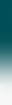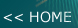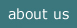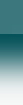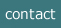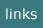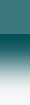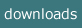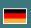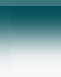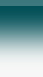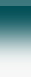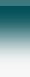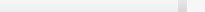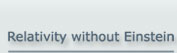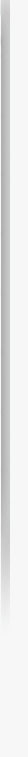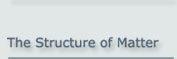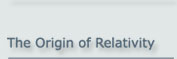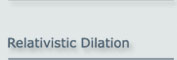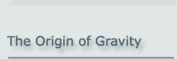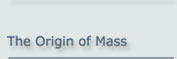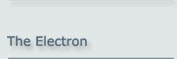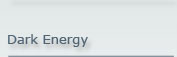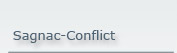The Origin of Mass
The Origin of Inertia

What is inertia?

Inertia is the property of matter
to set a force against a change of the actual speed
(amount and direction)
.

How is this force caused?

The force is caused by the binding fields inside an extended object.

How is an object extended?

The extension of an object is caused by the fact that the constituents of the object are bound to each other so as enforce a distance beween them. The forces which cause the bind are subject to the limitation of the speed of light like all forces. When such object is now in steady motion then the binding field will move with the object. That is a stable state in which the binding forces assure an invariant structure of the constituents. There are no external forces in this stable state.

What causes inertia?

If the constituents of an extended object are accelerated, the binding field follows the acceleration. But this happens with a delay, as the field is subject to the finiteness of the speed of light. This causes a temporary resistance against this acceleration.

The mechanism of this process will be described in the following.

_________________________________________________________________________

1. Summary

The origin of mass is one of the central unresolved questions in modern physics. For quite a long time physicists have been looking for a reason why matter should display inertial behaviour. The search for the Higgs boson is one example of this. However, the Higgs model is not the answer to this question as it will be shown.

There is a simple and very fundamental way to explain inertial mass. If two objects are bound to each other such that the binding field enforces a specific separation, then each time the position of one of them changes, a finite time elapses before the other particle moves, due to the finite speed of light. This delay is the cause of inertial behaviour.

From the above argument it turns out that the inertial mass of an elementary particle is given by the universal equation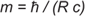where R is the radius of the particle. The validity of this equation can easily be checked for elementary particles carrying an electric charge. In such cases, the radius R follows classically from the magnetic moment, and when this radius is inserted into the above equation it yields precisely the correct mass!

This explanation of mass requires a model of an elementary particle in which it is composed of 2 sub-particles. Although such a model conflicts with currently accepted theoretical physics, it does not contradict any experimental observations, provided the model presented here is applied consistently.

Furthermore, the relativistic increase in mass due to motion and the resulting mass-energy formula (Einstein) are explained perfectly by this mechanism. -

Please note that the model presented here does not have any free parameters to be adjusted - which means to be fine-tuned.

(Note: This site is also available as a pdf file)

2. The Fundamental Mechanism of Inertial Mass

When a physical object has an extension, there can be two possible reasons:

1. The planetary case: The constituents each have a mass and orbit each other. The centrifugal inertial force balances the attractive force. Examples of this include the planetary orbits of the so-lar system and the Bohr model of the atom

2. The multipole case: The constituents are bound together while at the same time being kept apart by a multipole field originating in each of the constituents. A classical example of this is the individual atoms that make up a molecule.

Only the second alternative can be used in the case considered here, since we wish to explain the existence of mass in a configuration in which the basic constituents have no mass.

We will therefore investigate the situation of two fundamental ("basic") particles, which have no mass and which are bound to each other at a separation r0 . Such a bond at a distance is, as we have mentioned, realised by a multipole field, meaning that one particle (B) resides at the minimum of the potential of the field due to other particle (A) and vice versa, as shown in the figure 2.1. In each case, U is the binding potential.Figure 2.1:

The field of particles A and B bound to each other to maintain a separation r0

If one of the particles, e.g. particle A, is now held in a fixed position and the other, particle B, is moved towards or away from particle A by a certain amount Δr, the field tries to move particle B back to its original separation r0  from A. Hence a force must be applied to achieve this displacement.

Now consider a different situation. If neither of the particles is fixed and one particle, e.g. B, is moved by an external action, the other particle will follow. However, this will not happen instantaneously. The change in the field caused by the change in position of particle B can only propagate at the speed of light c. This means that for a time interval given by(2.1)

particle A is still held in its position by the “old” field of particle B, which has not yet changed. Also, the field due to particle A is unchanged at the position of particle B and therefore tries to keep particle B in its place. This means that a force is necessary to move particle B.

After a time Δt, the change in the field due to particle B will reach particle A, which will now move with the field to a position that corresponds to the new position of particle B. Consequently, after a further period of time Δt the field due to particle A at the position of particle B will adjust to the new position of particle A, and the force acting on the displaced particle B will disappear. Now both particles will be moving and no force will be necessary any longer.

A detailed step-by-step illustration of this process may be found here.

And an animation is additionally given at the  end of this site.

The particle model presented here is given the name “Basic Particle Model”.

3. Quantitative Determination of the Mass of an Elementary Particle

To calculate this effect quantitatively, it is necessary to know the structure of the field binding the two particles.

To determine the structure of this field we will use the approach that the binding field between the two particles should be the simplest type of multipole field, which fulfils the requirement of a well defined distance between the constituents. The following corresponds to that shown in the above diagram (figure 2.1):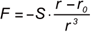or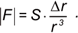(3.1)
where F is the force due to the field, S is the field constant, which associated with the strong force; r is the distance between the two particles in the multipole configuration, and r0 the equilibrium distance (corresponding to Δr = 0) at which the force disappears.

When we investigate the motion of this configuration, we will only consider small accelerations, where ‘small’ means that during a time Δt.the acceleration leads to a change in velocity, Δv ‹ ‹ c. For such changes, the denominator of (3.1) can be assumed to be constant over the time interval Δt.

We will now consider the case where the particle B is accelerated at a constant rate starting at a specific moment.
Then for a time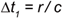(3.2)

after the onset of the acceleration, the field at the position of A will not change and A will therefore remain where it is. Immediately after this initial period, Δt1, the field change due to the motion of B will reach particle A which will then be accelerated constantly. The acceleration of particle A will follow the acceleration of particle B. On the other hand, the change in the field caused by the changing position of particle A will reach particle B after a further delay of Δt1. This delay leads to a constant, additional displacement of the fields between the particles, resulting in a constant force between them. - This situation is portrayed in figure 3.1Figure 3.1:

Configuration of the field at constant acceleration –
The dotted objects and lines show the parts and fields at rest, the solid objects and lines the
situation in motion

As a consequence of the constant acceleration a acting on B, particle B will have during the time 2Δt1 moved a distance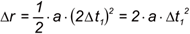(3.3)
which is now added to the equilibrium distance. According to eq. (3.1) the retarding force on particle B in the direction of motion, Fdm, will increase to the value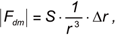(3.4)
where Δr is given by eq. (3.3).

So particle B will experience a constant force given by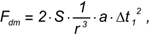(3.5)
or, using (3.2) for the time offset,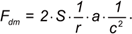(3.6)
According to Newton’s definition, inertial mass is given by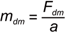and therefore the inertia in the direction of motion mdm is given by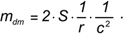(3.7)

Dependence on direction:

Returning to eq. (3.4), we must now take into account the fact that the full force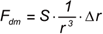is only effective in the moment when both basic particles are positioned on a line parallel to the direction of the applied force.

Due to their orbital motion, the basic particles are in reality positioned at varying angles to each other in relation to the direction of the external force, so that only part of this force is effective.

Rather than calculating now the integral over the various directions, we will at this point understand the field constant S so as to include the integration factor. This is possible as we will anyway identify the effective S by the use of known physical relations.

We will now determine the parameter S.

At first we will replace in eq. (3.7) the distance r by the radius R of the orbit: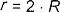So we get for (3.7)(3.8)

We will now use the magnetic moment of the electron to replace the radius R:(3.9)

This inserted into (3.8) and reordered yields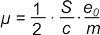(3.10)
This is a reproduction of the well-known Bohr magneton:which is normally deduced by quantum mechanics and well confirmed by measurement. So we get the value of S: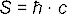(3.11)
which this (3.11) is now inserted into (3.8) the result is the quite simple formulaThis result has the following remarkable aspects:

1. It yields the fact that the quotient of force and acceleration is constant at non-relativistic velocities. Therefore this is a deduction of Newton’s law of motion. By Newton, this law was only postulated
2. The result shows that the mass is inversely proportional to the size of an elementary particle R.

The following further points should be noted:

1. The Bohr magneton was deduced here in a classical way following the Basic Particle Model. This is in contrast to the statement of textbooks that it can only be deduced on the basis of quantum mechanics
2. As it follows from the standard model of particle physics that this Bohr relation is valid in a corresponding way not only for all leptons but also for all quarks, the resulting equation for the particle mass (3.12) is valid for all elementary particles
3. deduction was done here by use of the electron. But one important fact was omitted. Only the internal bound in the electron was used which is realized by the strong force. The electric charge in the electron was not taken into account. This explains why the Bohr magneton is only an approximation for its magnetic moment. The correction for the electric charge, which is normally referred to as the Landé factor, is presented in the context of the description of the
4. electron.

4. The Relativistic Change of the Mass of an Elementary Particle

The increase in the mass of a moving body can also be deduced quite easily. In eq. (3.12), the radius, and hence the size, of the particle is in the denominator. If the particle now contracts as given by relativistic contraction: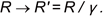(4.1)
where γ is the Lorentz factor:then an immediate result of this is that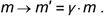(4.2)

Note:
It should be noted that this is an abbreviated explanation. If we look at the mechanism that underlies inertia, as given in chapter 3, there are further consequences. The delay by which the binding field is propagated increases, and the binding field itself changes in motion. However further analysis shows that these effects cancel each other out. Hence the simplified deduction presented above yields the correct result.

5. Mass Energy Equivalence

From eq. (4.6) (now adjusted for m→ m0 and m’→ m):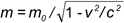or equivalently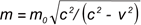(5.1)

it follows that an increase in the velocity of an object, which of course means an increase in its kinetic energy, will also increase its mass. The relationship between mass and energy, which is the most famous equation put forward by Einstein, will now be deduced quantitatively.

Eq. (5.1) is squared and rearranged to give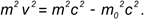Using the definition of momentum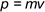it follows that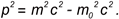Now the change in the momentum p resulting from a change in mass due to the particle’s motion is found by differentiation: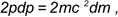which, again using p = mv, yields(5.2)
Energy is defined by Newton as follows: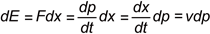(5.3)
If this definition of dE is inserted into eq. (5.2), it follows directly that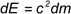(5.4)
which was Einstein’s original equation.
Integrating this and setting E=0 at m=0, we end up with the well-known result: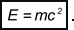(5.5)

Hence this famous and important formula can also be derived from the Basic Particle Model using conventional physics, whereas Einstein had to resort to Maxwell’s theory and perform a gedanken experiment using the momentum of a reflected light pulse to deduce this formula, which was origi-nally restricted to light.

Note:
Following up the idea that mass energy equivalence is simply a consequence of the structure of an elementary particle has a remarkable further consequence. The reverse conclusion may be drawn that mass energy equivalence may not be valid below the level of an elementary particle, i.e. energy mass equivalence and energy conservation may not apply to the constituents of an elementary parti-cle and to their interactions!

6. Angular Momentum of a Particle (Spin)

Equation (3.12) can be rearranged to yield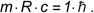(6.1)
The left-hand side is the classical definition of the angular momentum (spin) for v = c.

The right-hand side fulfils expectations towards the spin of an elementary particle in so far as it is independent of any particular properties of the particle; so its value is universal.

The factor 1 on the right-hand side is unsatisfactory at first glance because the measured spin incorporates a factor of ½. It should, however, not be a surprise. Eq. (6.1) gives the angular momentum for a system of two objects orbiting each other at a velocity c and separated by at a distance 2R, each representing half of the classical mass of the elementary particle. The configuration of the Basic Particle Model is, however, different in that the two objects do not have any classical mass. So, the deduction leading to (6.1) is a very formal one not taking into account specific properties of this model.

7
. Why not the Higgs Model?

By present main stream physics the Higgs Model is assumed to explain the inertia of elementary particles.

This is, however, not the case. There are two essential points which discard the Higgs model for the mass.

a.) Following the Higgs model, not the Higgs particle itself but the Higgs field, which is a vacuum field, is the cause of inertia. Now to explain the mass of the existing particles, the Higgs field has to have certain strength. On the other hand, the strength of a possible vacuum field can be limited by the observations in context with the expansion of the universe. The discrepancy between the necessary and the existing field is given by the following numbers [1,2]:
-  The necessary strength of a Higgs field is -(100 GeV)4 = -1044 eV
-  The vacuum field concluded from the cosmological constant is not more than 10-12 eV.

So the discrepancy between theory and observation amounts to a factor of at least 1056, and the field has the wrong sign.

b.) The mass mp of a specific particle is, according to the Higgs theory, given by the equation: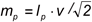where v is a universal field constant set to be 254 GeV/c2 and lp is the Yukawa coupling of that particle. This Yukawa coupling is, however, not given by the Higgs theory. It has to be determined individually for each particle by measurement.

That means that the Higgs theory is not able to give us the mass of any elementary particle.

8. Conclusion

If the structure of an elementary particle is as presented here and the binding field inside is as assumed regarding its shape, then this model of the origin of inertial mass provides an explanation of the inertial mass of elementary particles.

If an appropriate value for the strength of the binding field is taken from the evaluation of the Bohr magneton, then this model provides a correct and precise explanation of the mass of elementary particles.

The relativistic behaviour of the inertial mass as well as energy mass equivalence, Einstein’s most famous formula, are immediate consequences of this model. Furthermore, the constancy of spin and the correct value of the magnetic moment of a charged elementary particle are consequences of the Basic Model of Matter. Physics text books claim that these results can only be obtained using quantum mechanics. However the above derivation shows that they can in fact be understood classically on the basis of the Basic Particle Model.

And there is no need to assume further mechanisms to account for mass, such as the Higgs fields.

The concept of the Basic Model of Matter was first presented by Albrecht Giese at the Spring Conference of the German Physical Society (Deutsche Physikalische Gesellschaft) on 24 March 2000 in Dresden.

2021-05-16

 Steven D. Brass, The cosmological constant puzzle, Journal of Physics G, Nuclear and Particle Physics 38, 4(2011) 43201
 Frank J. Tipler, Genesis: How the Universe Began, arXiv:astro-ph/0111520v1 28 Nov 2001

Appendix

The Acceleration of a System of 2 Particles as a Step-by-Step Process

In the following, we will show in detail how the process of field transfer works, in order to give a better idea of why two particles located at a distance from each other display inertial behaviour. We start with the initial situation in which each particle is at rest, with no resultant force in the potential field of the other particle. Particle B (figure A.1) experiences no resultant force at the minimum of the potential due to A, which is symbolised by the relaxed spring on its right-hand side.Figure A.1:

Particles A and B in a force-free equilibrium state

In the next step, particle B is displaced to the right by a certain amount (figure A.2). This means that B is moved away from the potential minimum, so a force is required, which is depicted here by the stretching of the spring. (Remark: A sudden change in the position of B is assumed here, which is not physically accurate but helps to understand the process more easily.)Figure A.2:

Particle B pulled away from the potential minimum by a force

As a result of displacing B, the field due to B follows B to the right. This happens at the speed of light c. The change in the field requires a time interval Δt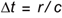(1)

to propagate from one particle to the other one.

So after a time ½ Δt, the change in the field has moved halfway to A (figure A.3).Figure A.3:

Displacement of field due to B has travelled half the way to A

Then, after a time 1 Δt , the change in the field reaches particle A (figure A.4). At this point, particle A instantaneously moves to the right, to the new position of minimum potential.Figure A.4:

Displacement of field due to B reaches A

A further consequence of this is that the field due to A also moves to the right. So, after a further time interval of ½ Δt, i.e. after 1½ Δt overall, it is has covered half the distance to B (figure A.5).Figure A.5:

Displacement of field due to A has covered half the distance to B

Finally, after a time 2 Δt, the repositioned field due to A reaches B, so that B is now once again at the minimum potential of the field due to A. This means no force is necessary any more to keep B in its new position as now represented by the relaxed spring (figure A.6).Figure A.6:

Displacement of field due to A reaches B

The full cycle of the changes of positions and fields shows, that an intermediate force is necessary in order to move this system of 2 particles to a new position.

In fact, the system is not only moved to a new position, but it is now in a state of motion. This is a consequence of the relativistic contraction of fields in motion. When the fields due to B and A move as a result of displacing the two particles, these fields contract. Hence at the end of the cycle both particles are located at a reduced distance r0' from each other. The new separation r0' is stable only if the configuration is moving and vice versa.

This phenomenon, whereby a force must be applied for an intermediate time in order to cause a change of the state of motion, is the physical phenomenon of inertial mass.

This process is demonstrated as an animation in the following:

Animation

The animation has 2 elements:

1. in  the first step the effect of a bind is demonstrated

2. in  the second step process of inertia is shown